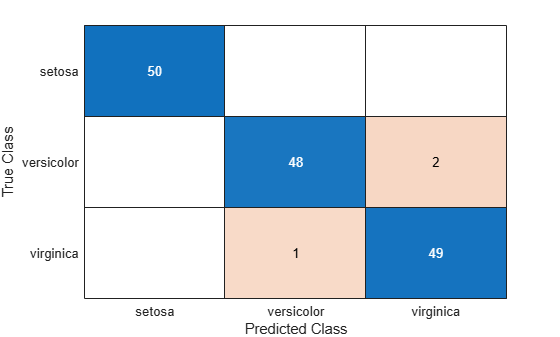# kfoldPredict

Predict response for observations not used for training

## Syntax

```label = kfoldPredict(obj) [label,score] = kfoldPredict(obj) [label,score,cost] = kfoldPredict(obj) ```

## Description

`label = kfoldPredict(obj)` returns class labels predicted by `obj`, a cross-validated classification. For every fold, `kfoldPredict` predicts class labels for in-fold observations using a model trained on out-of-fold observations.

```[label,score] = kfoldPredict(obj)``` returns the predicted classification scores for in-fold observations using a model trained on out-of-fold observations.

```[label,score,cost] = kfoldPredict(obj)``` returns misclassification costs.

## Input Arguments

 `obj`

## Output Arguments

 `label` Vector of class labels of the same type as the response data used in training `obj`. (The software treats string arrays as cell arrays of character vectors.) Each entry of `label` corresponds to a predicted class label for the corresponding row of `X`. `score` Numeric matrix of size `N`-by-`K`, where `N` is the number of observations (rows) in `obj``.X`, and `K` is the number of classes (in `obj.ClassNames`). `score(i,j)` represents the confidence that row `i` of `obj``.X` is of class `j`. For details, see More About. `cost` Numeric matrix of misclassification costs of size `N`-by-`K`. `cost(i,j)` is the average misclassification cost of predicting that row `i` of `obj``.X` is of class `j`.

## Examples

expand all

Find the cross-validation predictions for a model based on Fisher's iris data.

`load fisheriris`

Train an ensemble of classification trees using AdaBoostM2. Specify tree stumps as the weak learners.

```rng(1); % For reproducibility t = templateTree('MaxNumSplits',1); Mdl = fitcensemble(meas,species,'Method','AdaBoostM2','Learners',t);```

Cross validate the trained ensemble using 10-fold cross validation.

`CVMdl = crossval(Mdl);`

Estimate cross-validation predicted labels and scores.

`[elabel,escore] = kfoldPredict(CVMdl);`

Display the maximum and minimum scores of each class.

`max(escore)`
```ans = 1×3 9.3862 8.9871 10.1866 ```
`min(escore)`
```ans = 1×3 0.0018 3.8359 0.9573 ```

Create a confusion matrix using the 10-fold cross-validation predictions of a discriminant analysis model.

Load the `fisheriris` data set. `X` contains flower measurements for 150 different flowers, and `y` lists the species, or class, for each flower. Create a variable `order` that specifies the order of the classes.

```load fisheriris X = meas; y = species; order = unique(y)```
```order = 3x1 cell {'setosa' } {'versicolor'} {'virginica' } ```

Create a 10-fold cross-validated discriminant analysis model by using the `fitcdiscr` function. By default, `fitcdiscr` ensures that training and test sets have roughly the same proportions of flower species. Specify the order of the flower classes.

`cvmdl = fitcdiscr(X,y,'KFold',10,'ClassNames',order);`

Predict the species of the test set flowers.

`predictedSpecies = kfoldPredict(cvmdl);`

Create a confusion matrix that compares the true class values to the predicted class values.

`confusionchart(y,predictedSpecies)`